# QUESTION 1 A current of 2.00A was passed through sulfuric acid for 30 mins. How many...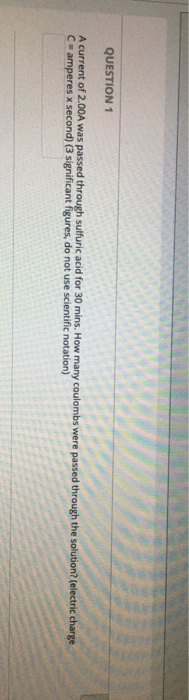QUESTION 1 A current of 2.00A was passed through sulfuric acid for 30 mins. How many coulombs were passed through the solution? (electric charge C amperes x second) (3 significant figures, do not use scientific notation)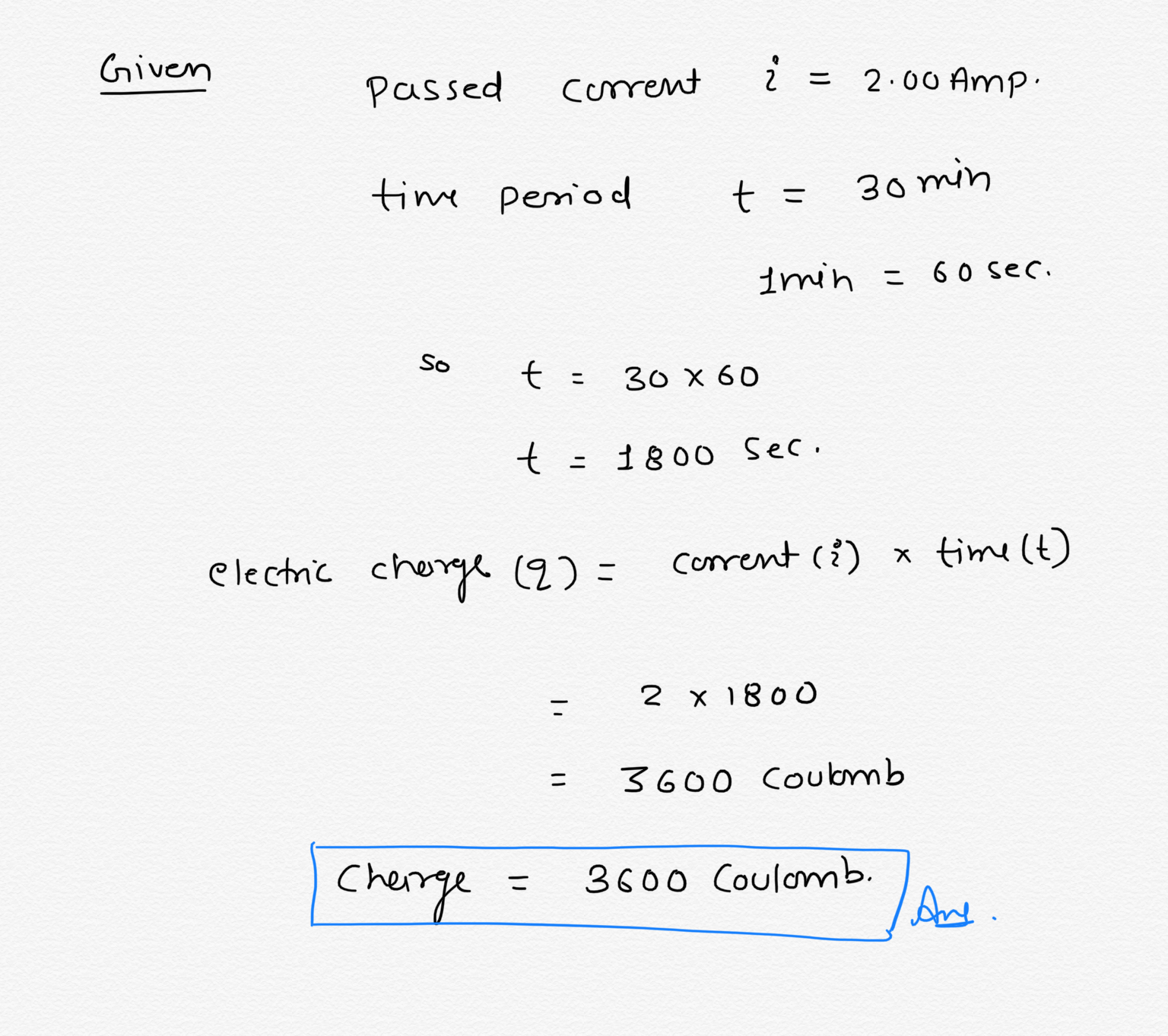#### Earn Coin

Coins can be redeemed for fabulous gifts.

Similar Homework Help Questions
• ### what are 17 and 18? Question 17 (10 points) A constant current is passed through a...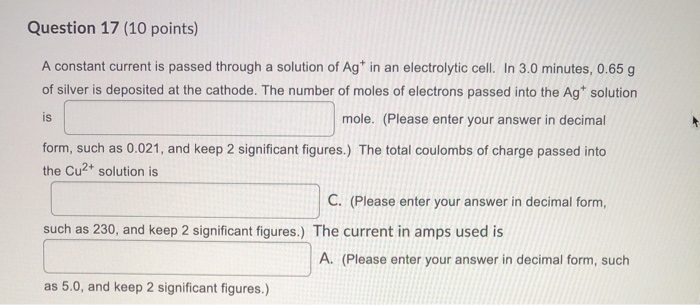what are 17 and 18? Question 17 (10 points) A constant current is passed through a solution of Agt in an electrolytic cell. In 3.0 minutes, 0.65 g of silver is deposited at the cathode. The number of moles of electrons passed into the Agt solution is mole. (Please enter your answer in decimal form, such as 0.021, and keep 2 significant figures.) The total coulombs of charge passed into the Cu2+ solution is C. (Please enter your answer in...

• ### Question 17 (10 points) Saved A constant current is passed through a solution of Agt in...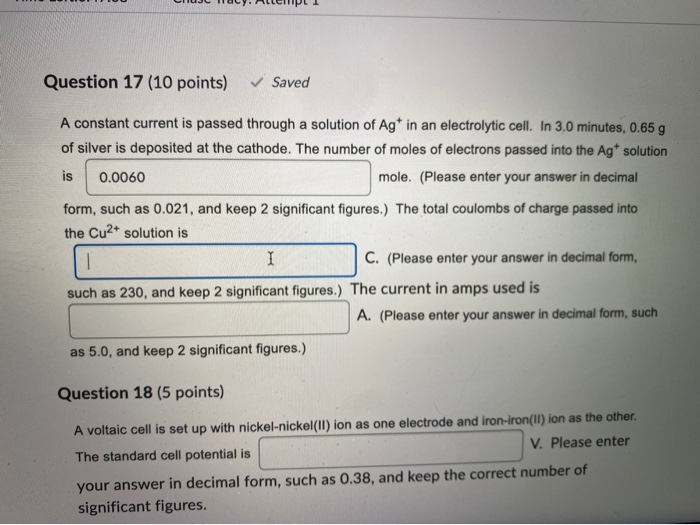Question 17 (10 points) Saved A constant current is passed through a solution of Agt in an electrolytic cell. In 3.0 minutes, 0.65 g of silver is deposited at the cathode. The number of moles of electrons passed into the Agt solution is 0.0060 mole. (Please enter your answer in decimal form, such as 0.021, and keep 2 significant figures. The total coulombs of charge passed into the Cu2+ solution is I C. (Please enter your answer in decimal form,...

• ### In a titration, 23.57 mL of 1.37 M NaOH were added to an acid. How many...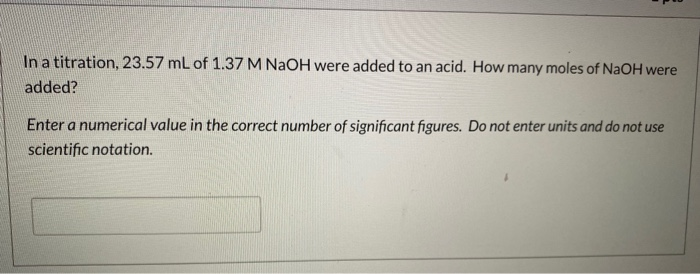In a titration, 23.57 mL of 1.37 M NaOH were added to an acid. How many moles of NaOH were added? Enter a numerical value in the correct number of significant figures. Do not enter units and do not use scientific notation. In a titration, 50.00 mL of 0.916 M H2SO4 were added. How many moles of H* were delivered? Enter a numerical value in the correct number of significant figures. Do not enter units and do not use scientific...

• ### QUESTION 15 How many moles of solute are in 544mL of 4M HNO3? • Answer to...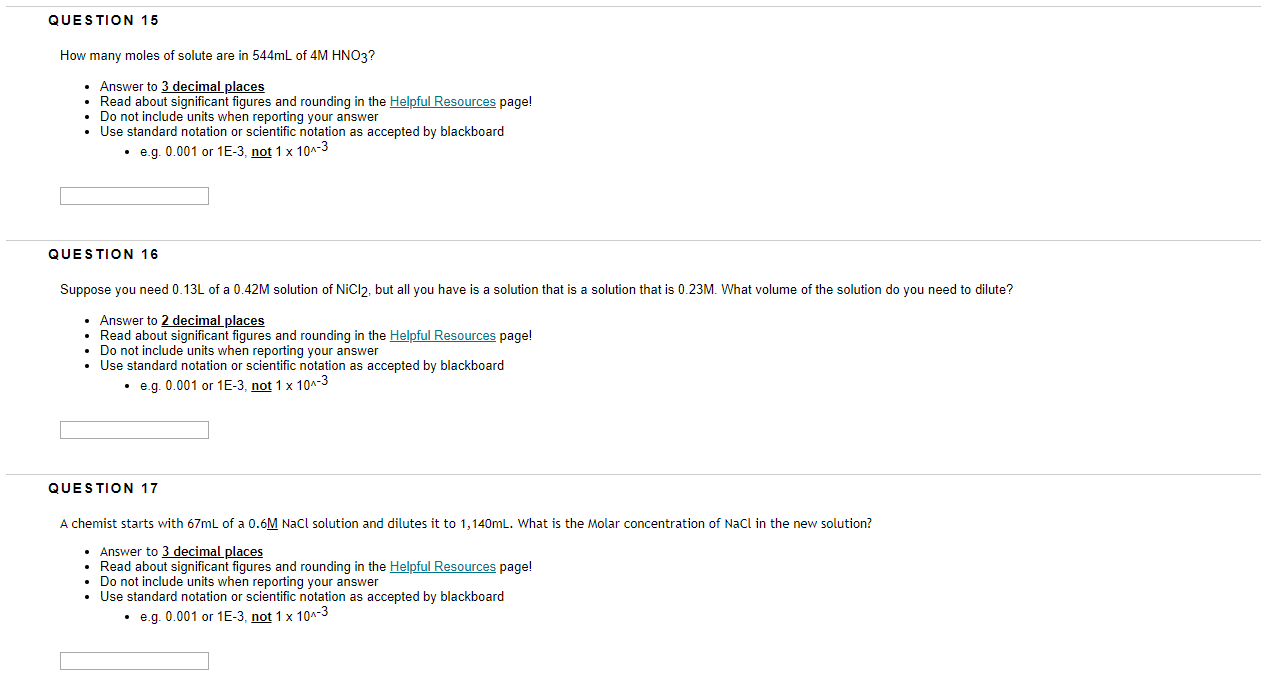QUESTION 15 How many moles of solute are in 544mL of 4M HNO3? • Answer to 3 decimal places • Read about significant figures and rounding in the Helpful Resources page! • Do not include units when reporting your answer • Use standard notation or scientific notation as accepted by blackboard . e.g. 0.001 or 1E-3, not 1 x 10^-3 QUESTION 16 Suppose you need 0.13L of a 0.42M solution of NiCl2, but all you have is a solution that...

• ### E.C. A constant current was passed through a solution of Cut2 for 5.00 min. The cathode...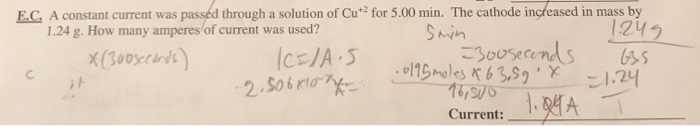E.C. A constant current was passed through a solution of Cut2 for 5.00 min. The cathode increased in mass by 1.24 g. How many amperes of current was used? smin 1.245 X 13008ccords ICELAIS 300 seconds 6.5 0195 moles & 63,59 X 2.506x10 1.24 16,510 Current: 1.04A 1

• ### When electricity (the flow of electrons) is passed through a solution, it causes an oxidation-reduction (redox)...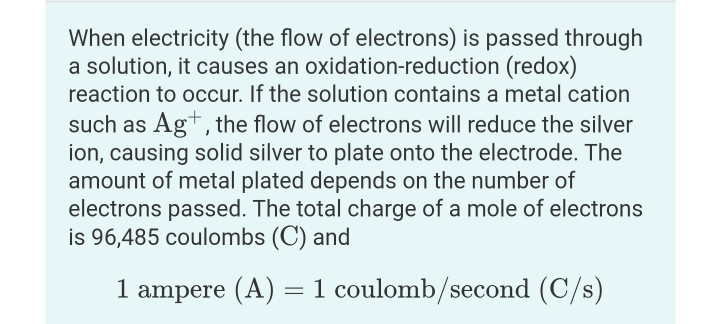When electricity (the flow of electrons) is passed through a solution, it causes an oxidation-reduction (redox) reaction to occur. If the solution contains a metal cation such as Ag+, the flow of electrons will reduce the silver ion, causing solid silver to plate onto the electrode. The amount of metal plated depends on the number of electrons passed. The total charge of a mole of electrons is 96,485 coulombs (C) and 1 ampere (A) = 1 coulomb/second (C/s) Part A...

• ### How many atoms of hydrogen are in 2.22E+1 g of methane? Respond with the correct number...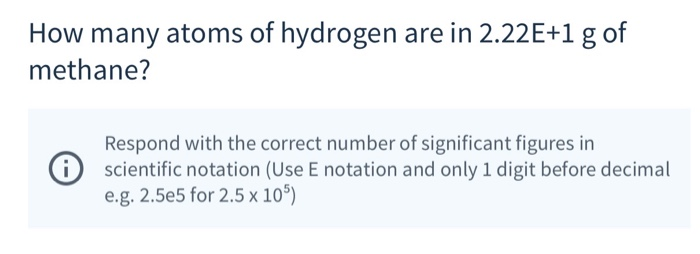How many atoms of hydrogen are in 2.22E+1 g of methane? Respond with the correct number of significant figures in scientific notation (Use E notation and only 1 digit before decimal e.g. 2.5e5 for 2.5 x 10") How many moles of oxygen atoms are in 5.3E-1 moles of CO2? Only give the numeric value of your answer. Don't include the units. .- Respond with the correct number of significant figures in scientific notation (Use E notation and only 1 digit...

• ### In a 2.86E+1 g sample of NaHCO3, how many grams of sodium are present? Use two...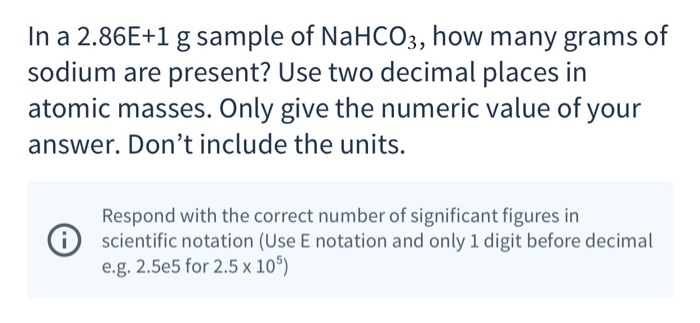In a 2.86E+1 g sample of NaHCO3, how many grams of sodium are present? Use two decimal places in atomic masses. Only give the numeric value of your answer. Don't include the units. i Respond with the correct number of significant figures in scientific notation (Use E notation and only 1 digit before decimal e.g. 2.5e5 for 2.5 x 105) In a 1.018E+2 g sample of iron(111) oxide, how many grams of iron are present? Your answer should have the...

• ### Part B and D Please show all of the steps. Learning Goal: To relate current, time,...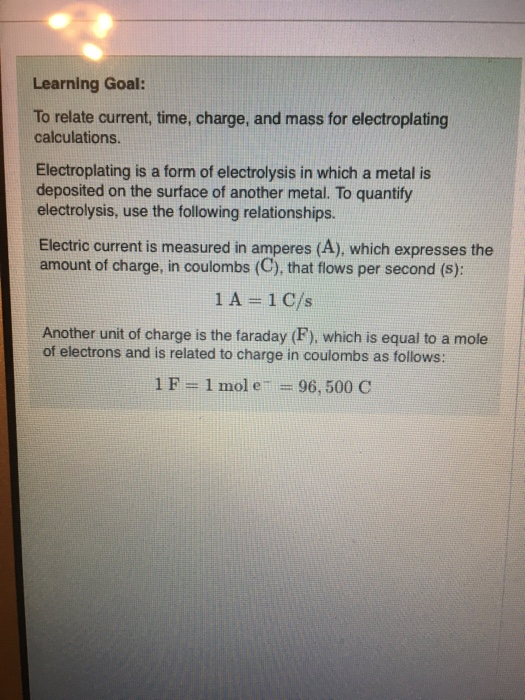Part B and D Please show all of the steps. Learning Goal: To relate current, time, charge, and mass for electroplating calculations. Electroplating is a form of electrolysis in which a metal is deposited on the surface of another metal. To quantify electrolysis, use the following relationships. Electric current is measured in amperes (A), which expresses the amount of charge, in coulombs (C), that flows per second (s): 1 A 1 C/s Another unit of charge is the faraday (F),...

• ### 43. If a 2.5 A current flows through a circuit for 35 minutes, how many coulombs...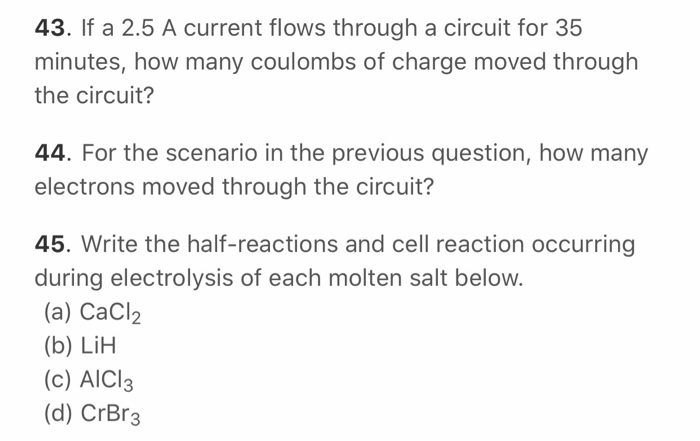43. If a 2.5 A current flows through a circuit for 35 minutes, how many coulombs of charge moved through the circuit? 44. For the scenario in the previous question, how many electrons moved through the circuit? 45. Write the half-reactions and cell reaction occurring during electrolysis of each molten salt below. (a) CaCl2 (b) LIH (c) AICI3 (d) CrBr3